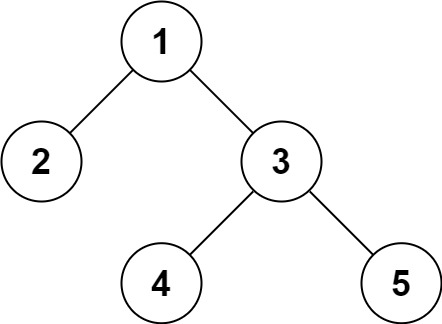# 297.serialize-and-deserialize-binary-tree

## Statement

• Difficulty: Hard
• Tag: `树` `深度优先搜索` `广度优先搜索` `设计` `字符串` `二叉树```````输入：root = [1,2,3,null,null,4,5]

``````

``````输入：root = []

``````

``````输入：root = 

``````

``````输入：root = [1,2]

``````

• 树中结点数在范围 `[0, 104]`
• `-1000 <= Node.val <= 1000`

• Link: Serialize and Deserialize Binary Tree
• Difficulty: Hard
• Tag: `Tree` `Depth-First Search` `Breadth-First Search` `Design` `String` `Binary Tree`

Serialization is the process of converting a data structure or object into a sequence of bits so that it can be stored in a file or memory buffer, or transmitted across a network connection link to be reconstructed later in the same or another computer environment.

Design an algorithm to serialize and deserialize a binary tree. There is no restriction on how your serialization/deserialization algorithm should work. You just need to ensure that a binary tree can be serialized to a string and this string can be deserialized to the original tree structure.

Clarification: The input/output format is the same as how LeetCode serializes a binary tree. You do not necessarily need to follow this format, so please be creative and come up with different approaches yourself.

Example 1:``````Input: root = [1,2,3,null,null,4,5]
Output: [1,2,3,null,null,4,5]
``````

Example 2:

``````Input: root = []
Output: []
``````

Constraints:

• The number of nodes in the tree is in the range `[0, 104]`.
• `-1000 <= Node.val <= 1000`

## Solution

``````# Definition for a binary tree node.
# class TreeNode(object):
#     def __init__(self, x):
#         self.val = x
#         self.left = None
#         self.right = None

class Codec:

def serialize(self, root):
"""Encodes a tree to a single string.

:type root: TreeNode
:rtype: str
"""
res = []

def dfs(rt):
if not rt:
res.append(str(1001))
return
res.append(str(rt.val))
dfs(rt.left)
dfs(rt.right)

dfs(root)

return ",".join(res)

def deserialize(self, data):
"""Decodes your encoded data to tree.

:type data: str
:rtype: TreeNode
"""
data = data.split(",")
ix = [-1]

def dfs():
ix += 1
if ix >= len(data):
return None

cur = int(data[ix])
if cur == 1001:
return None

t = TreeNode()
t.val = cur
t.left = dfs()
t.right = dfs()
return t

return dfs()

# Your Codec object will be instantiated and called as such:
# ser = Codec()
# deser = Codec()
# ans = deser.deserialize(ser.serialize(root))
``````